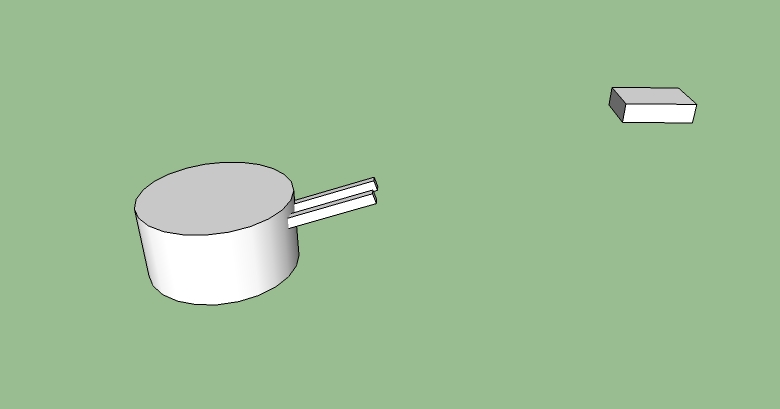CS 420 Game Engineering 3D
Final Practice Problems
1. You want to point your camera at an object.  You want the camera be as close as possible to "right side up", given that it is pointed at the object.  That is, you want the camera's up vector to be as close as possible to the world up vector, given that the camera is pointed directly at the object.  If the object has the position po = [pox, poy, poz], and the camera has the position pc = [pcx, pcy, pcz], give the rotational matrix for the camera.

2. You want to aim a turret.  The turret consists of two objects:  The "base", which can rotate around the y axis, and the barrel, which can be raised or lowered:The barrel is attached to the turret (so that it moves when the turret moves).  The barrel is attached at an offset of [0,0,0] in turret space (so the origin of the barrel is the same as the origin of the turret).  Assume that if the rotational matrix of the barrel (in turret space) is the identity matrix I, the barrel points straight down the z-axis of the turret.

You wish to rotate the turret and raise the barrel so that it points at an object at position p = [px, py, px].   Show how to create the rotational matrix for both the turret (in world space) and the barrel (in turret space).   Feel free to define "local variables" in your answer (such as, let x = || p1 × p2||)

3. You are representing planets in a solar system using the following scheme:
• The position of the sun us given a s a 3-Vector in world space possun
• The location of planet p1 is given as a scalar distance from the sun dp1 and orientation (quaternion) qp1 which describes how much we need to rotate the vector [0, 0, dp1] so that it points from the sun to planet p1
• Likewise, the location of planet p2 is given as a scalar distance from the sun dp2 and orientation (quaternion) qp2 which describes how much we need to rotate the vector [0, 0, dp2] so that it points from the sun to planet p2
• The location of moon m1 is given as a scalar distance from the planet p1, dm1 and orientation (quaternion) qm1 which describes how much we need to rotate the vector [0, 0, dm1] so that it points from the planet p11
• The location of moon m2 is given as a scalar distance from the planet p2, dm2 and orientation (quaternion) qm2 which describes how much we need to rotate the vector [0, 0, dm2] so that it points from the planet p22
Give an equation that describes the scalar distance between moon m1 and moon m2 as a function of possun, dp1, qp1,dp2, qp2, dm1, qm1,dm2 and qm2,
4. What is the output of the following fragment of C++ code:
```int &weird(int &x)
{
return ++x;
}

int main()
{
int x = 1;
int &y = weird(x);
printf("x = %d, y = &d \n", x, y);
y++;
printf("x = %d, y - %d \n", x, y);
}
```
Bonus question:  If we change the ++x to a x++, then the code will no longer compile.  Why not?

5. What is the output of the following fragment of lua code:
1. ```MyTable = { = 2,  = 2,  = 1,  = 1}
s1 = 0
s2 = 0
for k,v in pairs(MyTable) do
s1 = s1 + k
s2 = s2 + v
end
print(s1,s2)
```
2. ```x = 2
y = 3
function twist()
local x = 7
x,y = y, x
print(x,y)
end
twist()
print(x,y)
```
3. ```ft = {}
ft  = function(x, y) return y,x end
ft  = function(x, y, z) return z, y, x end
a, b, c = ft(1,2,3)
d, e, f = ft(4,5,6)
print(a, b, c)
print(d, e, f)
```